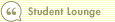Course 404: Putting DCF into Action
Step 4--Calculate Discounted Perpetuity ValueIn this course1 Introduction 2 Step 1--Project Free Cash Flow 3 Step 2--Determine a Discount Rate 4 Step 3--Discount Projected Free Cash Flows to Present 5 Step 4--Calculate Discounted Perpetuity Value 6 Step 5--Add It All Up 7 The Bottom LineIn this step, we use another formula from the last lesson:

Perpetuity Value =
( CFn x (1+ g) ) / (R - g)

CFn = Cash Flow in the Last Individual Year Estimated, in this case Year 10 cash flow
g = Long-Term Growth Rate
R = Discount Rate, or Cost of Capital, in this case cost of equity

For example, we'll use use 3% as the perpetuity growth rate, which is close to the historical average growth rate of the U.S. economy. So, we'll assume that after 10 years, Charlie's Bicycles will also grow at this 3% rate. Plugging the numbers into the formula:

( \$1,284 x (1 + .03) ) / (.09 - .03) = \$22,042 million

Notice that for the cash flow figure we used the undiscounted Year 10 cash flow, not the discounted \$542 million. But because we used the undiscounted amount, we still need to express the perpetuity value in present-value terms using this trusty formula:

Present Value of Cash Flow in Year N =
CF at Year N / (1 + R)^N

Present Value of Perpetuity Value =
\$22,042 million / (1 + .09)^10 = \$9,311 million

Next: Step 5--Add It All Up >>Search Print Lesson  | FeedbackDel.icio.us |digg itLearn how to invest like a pro with Morningstar’s Investment Workbooks (John Wiley & Sons, 2004, 2005), available at online bookstores.© Copyright 2015 Morningstar, Inc. All rights reserved. Please read our Privacy Policy. If you have questions or comments please contact Morningstar.